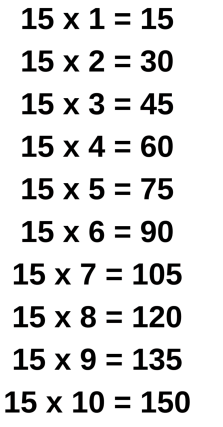# 15 Times Table- Learn Table Of 15 : Multiplication Table Of 15

Safalta expert Published by: Yashaswi More Updated Wed, 02 Mar 2022 10:15 PM IST

## Highlights

Check out how to learn the 15 Times table easily here at Safalta.com

Students must memorize the multiplication table of 15 at their fingertips. Learning 15 times table has a positive benefit including greater confidence and better results in doing arithmetic calculations. 15 times table is a multiplication table that consists of the multiplication of 15 with whole numbers. Before we begin to learn about it, we should know how to say the table of 15. Here it is! 15 ones are 15, 15 twos are 30, 15 threes are 45, 15 fours are 60, and so on. Join Safalta School Online and prepare for Board Exams under the guidance of our expert faculty.

### Free Demo ClassesSource: Safalta.com

Our online school aims to help students prepare for Board Exams by ensuring that students have conceptual clarity in all the subjects and are able to score their maximum in the exams.

1. Multiplication table of 15 chart
2.  Multiplication table of 15
3. 15 times table
4. Tips for 15 times table
5. Table of 15 upto 20

### Multiplication Table of 15 Chart:Let us learn in detail about the 15 times table and its applications.

## Multiplication Table of 15

Multiplication table of 15 is easy to learn. It is generated by multiplying the number 15 with all the natural numbers such as 15 ones are 15, mathematically it is written as 15 × 1 = 15. Similarly, 15 twos are 30. In math, it is written as 15 × 2 = 30. Here 15 and 30 are the multiples of 15.

Given below is a 15 times table. Go through the 15 times table for fast calculations and learn few more multiples of 15.

## Tips for 15 Times Table

1. To memorize the table of 15, there is a small trick. To learn this trick you should know about one's place digits, ten's place digits, odd numbers, and even numbers. The trick is that in the resultants, the one's place always follows the pattern of 5-0.

• 15 × 1 = 15
• 15 × 2 = 30

For ten's place digit in resultants, follow two consecutive even numbers and next two consecutive odd numbers.

• 15 × 1 = 15 (odd number at ten's place)
• 15 × 2 = 30 (odd number at ten's place)
• 15 × 3 = 45 (even number at ten's place)
• 15 × 4 = 60 (even number at ten's place)

## Table of 15 up to 20

Here is a 15 times table up to 20. Try to find a similar pattern here.

## Table of 15 Worksheet

1. ### Example 1: Using 15 times table, Evaluate: a) 15 times 8, b) 15 times 7 minus 5, and c) 6 times 6 plus 15 times 7

Solution:

a) First, we will write 15 times 8 mathematically.

Using 15 times table, we have 15 times 8 = 15 × 8 = 120.

Hence, 15 times 8 is 120.

b) First, we will write 15 times 7 minus 5 mathematically.

Using table of 15, we have 15 times 7 minus 5 = 15 × 7 - 5 = 105 - 5 = 100

Hence, 15 times 7 minus 5 is 100.

c) First, we will write 6 times 6 plus 15 times 7 mathematically.

Using table of 15, we have: 6 times 6 plus 15 times 7 = 6 × 6 + 15 × 7 = 36 + 105 = 141

Hence, 6 times 6 plus 15 times 7 is 141.

2. ### Example 2: Using 15 times table, find: a) What is the 19th multiple of 15? and b) What is the 15th multiple of 15?

Solution:

a) To find the 19th multiple of 15, we need to call out 15 times table till 19 or multiply 15 by 19. We get 15 × 19 = 285

Thus, the nineteenth multiple of 15 is 285.

b) Similarly, to find the 15th multiple of 1,5 we need to call out the table of 15 till 15 or multiply 15 by 15 i.e. 15 × 15 = 225

Thus, the fifteenth multiple of 15 is 225.

## Multiplication Tables

 2 Times Table 11 Times Table 3 Times Table 12 Times Table 4 Times Table 13 Times Table 5 Times Table 14 Times Table 6 Times Table 16 Times Table (Soon) 7 Times Table 17 Times Table (Soon) 8 Times Table 18 Times Table (Soon) 9 Times Table 19 Times Table (Soon) 10 Times Table 20 Times Table (Soon)

## What is the importance of learning Table 2 to 20?

For making mathematical section easier, memorising table 2 to 20 is important.

## How can I learn tables easily?

Daily recite the table as mentioned in the article twice and thrice.

## What is the 15 times table?

Using 15 times table, we have 15 times 8 = 15 × 8 = 120. Hence, 15 times 8 is 120. b) First, we will write 15 times 7 minus 5 mathematically.

## What are the multiples of 15?

Here is the list of all multiples: 15, 30, 45, 60, 75, 90, 105, 120, 135, 150, 165, 180, 195, 210, 225, 240, 255, 270, 285, 300, 315, 330, 345, 360, 375, 390, 405, 420, 435, 450, 465, 480, 495, 510, 525, 540, 555, 570, 585, 600, 615, 630, 645, 660, 675, 690, 705, 720, 735,

## What times what will give you 15?

1 x 15 = 15. 3 x 5 = 15. 5 x 3 = 15. 15 x 1 = 15.